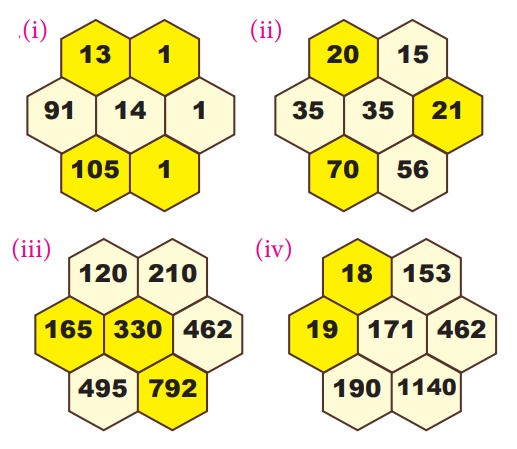Home | | Maths 7th Std | Exercise 5.2 (PascalŌĆÖs Triangle)

# Exercise 5.2 (PascalŌĆÖs Triangle)

7th Maths : Term 2 Unit 5 : Information Processing : PascalŌĆÖs Triangle : Exercise 5.2

Exercise 5.2

1. Complete the PascalŌĆÖs Triangle.Solution:2. The following hexagonal shapes are taken from PascalŌĆÖs Triangle. Fill in the missing numbers.Solution:3. Complete the PascalŌĆÖs Triangle by taking the numbers 1,2,6,20 as line of symmetry.Solution:4. The elements along the sixth row of the PascalŌĆÖs Triangle is

(i) 1,5,10,5,1

(ii) 1,5,5,1

(iii) 1,5,5,10,5,5,1

(iv) 1,5,10,10,5,1

Answer: (iv) 1, 5, 10, 10, 5, 1

5. The difference between the consecutive terms of the fifth slanting row containing four elements of a PascalŌĆÖs Triangle is

(i) 3,6,10,ŌĆ”

(ii) 4,10,20,ŌĆ”

(iii) 1,4,10,ŌĆ”

(iv) 1,3,6,ŌĆ”

6. What is the sum of the elements of nineth row in the PascalŌĆÖs Triangle?

(i) 128

(ii) 254

(iii) 256

(iv) 126

Exercise 5.2

1.2.3.Objective type questions

4. (iv) 1,5,10,10,5,1

5. (ii) 4,10,20,...

6. (iii) 256

Tags : Information Processing | Term 2 Chapter 5 | 7th Maths , 7th Maths : Term 2 Unit 5 : Information Processing
Study Material, Lecturing Notes, Assignment, Reference, Wiki description explanation, brief detail
7th Maths : Term 2 Unit 5 : Information Processing : Exercise 5.2 (PascalŌĆÖs Triangle) | Information Processing | Term 2 Chapter 5 | 7th Maths